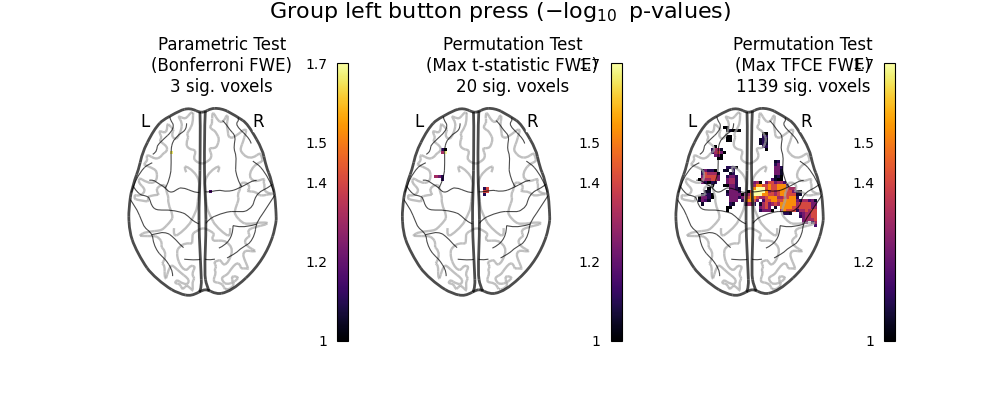# Massively univariate analysis of a motor task from the Localizer dataset#

This example shows the results obtained in a massively univariate analysis performed at the inter-subject level with various methods. We use the [left button press (auditory cue)] task from the Localizer dataset and seek association between the contrast values and a variate that measures the speed of pseudo-word reading. No confounding variate is included in the model.

1. A standard ANOVA is performed. Data smoothed at 5 voxels FWHM are used.

2. A permuted Ordinary Least Squares algorithm is run at each voxel. Data smoothed at 5 voxels FWHM are used.

Note

If you are using Nilearn with a version older than `0.9.0`, then you should either upgrade your version or import maskers from the `input_data` module instead of the `maskers` module.

That is, you should manually replace in the following example all occurrences of:

```from nilearn.maskers import NiftiMasker
```

with:

```from nilearn.input_data import NiftiMasker
```
```# Author: Virgile Fritsch, <virgile.fritsch@inria.fr>, May. 2014
import matplotlib.pyplot as plt
import numpy as np
from nilearn import datasets
from nilearn.mass_univariate import permuted_ols
```

```n_samples = 94
localizer_dataset = datasets.fetch_localizer_contrasts(
["left button press (auditory cue)"],
n_subjects=n_samples,
legacy_format=False,
)

# print basic information on the dataset
print(
"First contrast nifti image (3D) is located "
f"at: {localizer_dataset.cmaps}"
)

tested_var = localizer_dataset.ext_vars["pseudo"]

# Quality check / Remove subjects with bad tested variate
contrast_map_filenames = [
]
print(f"Actual number of subjects after quality check: {int(n_samples)}")
```
```First contrast nifti image (3D) is located at: /home/yasmin/nilearn/nilearn_data/brainomics_localizer/brainomics_data/S01/cmaps_LeftAuditoryClick.nii.gz
Actual number of subjects after quality check: 89
```

```nifti_masker = NiftiMasker(
smoothing_fwhm=5, memory="nilearn_cache", memory_level=1
)
```

Anova (parametric F-scores)

```from sklearn.feature_selection import f_regression

_, pvals_anova = f_regression(fmri_masked, tested_var, center=True)
pvals_anova[np.isnan(pvals_anova)] = 1
pvals_anova[pvals_anova > 1] = 1
neg_log_pvals_anova = -np.log10(pvals_anova)
neg_log_pvals_anova
)
```
```/home/yasmin/miniconda3/envs/nilearn-dev/lib/python3.10/site-packages/sklearn/utils/validation.py:1141: DataConversionWarning:

A column-vector y was passed when a 1d array was expected. Please change the shape of y to (n_samples, ), for example using ravel().
```

Perform massively univariate analysis with permuted OLS

This method will produce both voxel-level FWE-corrected -log10 p-values and TFCE-based FWE-corrected -log10 p-values.

Note

`permuted_ols` can support a wide range of analysis designs, depending on the `tested_var`. For example, if you wished to perform a one-sample test, you could simply provide an array of ones (e.g., `np.ones(n_samples)`).

```ols_outputs = permuted_ols(
tested_var,  # this is equivalent to the design matrix, in array form
model_intercept=True,
tfce=True,
n_perm=200,  # 200 for the sake of time. Ideally, this should be 10000.
verbose=1,  # display progress bar
n_jobs=1,  # can be changed to use more CPUs
output_type="dict",
)
ols_outputs["logp_max_t"][0, :]  # select first regressor
)
ols_outputs["logp_max_tfce"][0, :]  # select first regressor
)
```
```[Parallel(n_jobs=1)]: Using backend SequentialBackend with 1 concurrent workers.
Job #1, processed 0/200 permutations (0.00%, 9353.795051574707 seconds remaining)
Job #1, processed 10/200 permutations (5.00%, 194.07732915878296 seconds remaining)
Job #1, processed 20/200 permutations (10.00%, 177.8453986644745 seconds remaining)
Job #1, processed 30/200 permutations (15.00%, 164.5167976220449 seconds remaining)
Job #1, processed 40/200 permutations (20.00%, 153.1584596633911 seconds remaining)
Job #1, processed 50/200 permutations (25.00%, 142.51711463928223 seconds remaining)
Job #1, processed 60/200 permutations (30.00%, 132.99475900332135 seconds remaining)
Job #1, processed 70/200 permutations (35.00%, 124.26879034723555 seconds remaining)
Job #1, processed 80/200 permutations (40.00%, 114.65044248104095 seconds remaining)
Job #1, processed 90/200 permutations (45.00%, 104.6836956606971 seconds remaining)
Job #1, processed 100/200 permutations (50.00%, 95.05466508865356 seconds remaining)
Job #1, processed 110/200 permutations (55.00%, 85.53673865578392 seconds remaining)
Job #1, processed 120/200 permutations (60.00%, 76.30273214975992 seconds remaining)
Job #1, processed 130/200 permutations (65.00%, 67.11407151589026 seconds remaining)
Job #1, processed 140/200 permutations (70.00%, 57.65356319291251 seconds remaining)
Job #1, processed 150/200 permutations (75.00%, 47.94999329249064 seconds remaining)
Job #1, processed 160/200 permutations (80.00%, 38.22868353128433 seconds remaining)
Job #1, processed 170/200 permutations (85.00%, 28.62666245067821 seconds remaining)
Job #1, processed 180/200 permutations (90.00%, 19.10380416446262 seconds remaining)
Job #1, processed 190/200 permutations (95.00%, 9.543440994463468 seconds remaining)
[Parallel(n_jobs=1)]: Done   1 out of   1 | elapsed:  3.2min finished
```

Visualization

```from nilearn import plotting
from nilearn.image import get_data

# Various plotting parameters
z_slice = 12  # plotted slice
threshold = -np.log10(0.1)  # 10% corrected

vmax = max(
np.amax(ols_outputs["logp_max_t"]),
np.amax(neg_log_pvals_anova),
np.amax(ols_outputs["logp_max_tfce"]),
)

images_to_plot = {
"Permutation Test\n(Max t-statistic FWE)": (
),
}

fig, axes = plt.subplots(figsize=(12, 3), ncols=3)
for i_col, (title, img) in enumerate(images_to_plot.items()):
ax = axes[i_col]
n_detections = (get_data(img) > threshold).sum()
new_title = f"{title}\n{n_detections} sig. voxels"

plotting.plot_glass_brain(
img,
colorbar=True,
vmax=vmax,
display_mode="z",
plot_abs=False,
cut_coords=,
threshold=threshold,
figure=fig,
axes=ax,
)
ax.set_title(new_title)

fig.suptitle(
"Group left button press (\$-\\log_{10}\$ p-values)",
y=1.3,
fontsize=16,
)
``````Text(0.5, 1.3, 'Group left button press (\$-\\log_{10}\$ p-values)')
```

Total running time of the script: ( 3 minutes 22.115 seconds)

Estimated memory usage: 61 MB

Gallery generated by Sphinx-Gallery